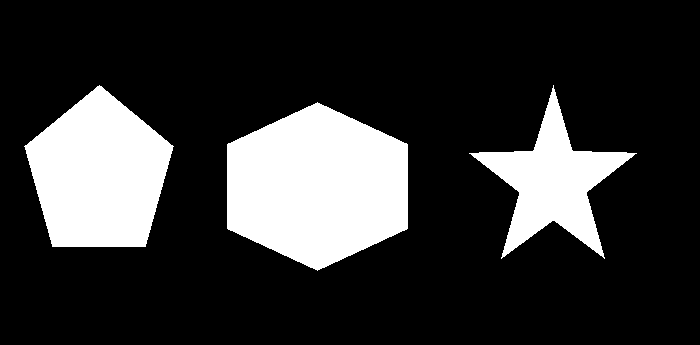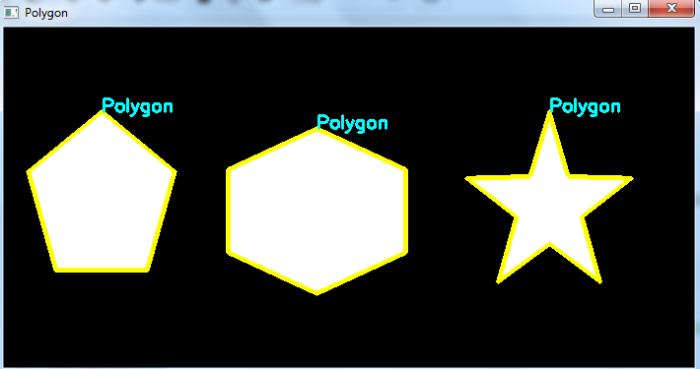# How to detect polygons in image using OpenCV Python?

We first detect all the object contours in the image to detect a polygon. Then Loop over all contours. Find the approximate contour for each of the contours. If the number of vertex points in the approximate contour is 5 or more then draw the contour and set it as a triangle. See the below pseudocode.

for cnt in contours:
approx = cv2.approxPolyDP()
if len(approx) >= 5:
cv2.drawContours()
cv2.putText("Polygon")


## Steps

We could use the following steps to detect polygons in an image −

• Import the required library. In all the following examples, the required Python library is OpenCV. Make sure you have already installed it.

• Read the input image using cv2.imread() and convert it to grayscale.

• Apply thresholding cv2.threshold() on the grayscale image to create a binary image. Adjust the second parameter to get a better contour detection.

• Find the contours in the image using cv2.findContours() function.

• Select a contour (say first contour) cnt from the lists of contours. Or Loop over all the detected contours.

• Compute the approximate contour points (approx) for each contour cnt using cv2.approxPolyDP() function.

• If the total number of vertex points in the approximate contour approx is 5 or more, then draw the approximate contours on the image and set it a polygon.

• Display the image with drawn contours and approximate contours on it.

Let's have a look at the example below for a better understanding.

## Example

In this Python program, we detect the polygons in the input image. We also draw the contours of the detected polygons.

# import required libraries
import cv2

# convert the image to grayscale
gray = cv2.cvtColor(img, cv2.COLOR_BGR2GRAY)

# apply thresholding to convert the grayscale image to a binary image
ret,thresh = cv2.threshold(gray,50,255,0)

# find the contours
contours,hierarchy = cv2.findContours(thresh, cv2.RETR_TREE,cv2.CHAIN_APPROX_SIMPLE)
print("Number of contours detected:",len(contours))
for cnt in contours:
approx = cv2.approxPolyDP(cnt, 0.01*cv2.arcLength(cnt, True), True)
(x,y)=cnt[0,0]

if len(approx) >= 5:
img = cv2.drawContours(img, [approx], -1, (0,255,255), 3)
cv2.putText(img, 'Polygon', (x, y),
cv2.FONT_HERSHEY_SIMPLEX, 0.6, (255, 255, 0), 2)
cv2.imshow("Polygon", img)
cv2.waitKey(0)
cv2.destroyAllWindows()


We will use the following image as the Input File for this program −When you run the above Python program, it will produce the following output window −

Number of contours detected: 3


And we get the following output window −